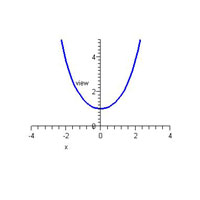MERLOT Mathematics Portal Visit Main MERLOT# Mathematical Curve Conjectures

This material is replicated on a number of sites as part of the SERC Pedagogic Service Project

## Summary

In this activity, a six-foot length of nylon rope is suspended at both ends to model a mathematical curve known as the hyperbolic cosine. In a write-pair-share activity, students are asked to make a conjecture concerning the nature of the curve and then embark on a guided discovery in which they attempt to determine a precise mathematical description of the curve using function notation.## Learning Goals

To enable students to:

• develop the skill of making and verifying mathematical conjectures
• develop the skill of mathematical modeling
• gain a deeper understanding of the hyperbolic cosine (hanging curve) function
• recognize the differences between the quadratic and hyperbolic cosine curves

## Context for Use

This activity can be carried out in either a small class or a large lecture setting. It is best presented as an introduction to the hyperbolic functions normally studied near the end of Calculus I or near the beginning of Calculus II. This project requires students to apply what they have learned about functions to a real-world scenario, viz., a suspended rope, in an attempt to construct an accurate mathematical model.

The activity takes approximately 30 minutes although less time may be taken if desired.

## Description and Teaching Materials

Materials

• Six-foot length of 3/8" nylon rope
• Sliding whiteboard/blackboard clips or tape to attach the rope to a whiteboard or blackboard
• Rectangular grid board or underlay (this may be constructed directly on the blackboard or whiteboard if necessary with the use of a ruler and chalk or marker)
• Overhead or LCD projector to display superimposed graphs of y=x^2+1 and y=cosh(x)

Activity description

• The instructor suspends the rope from movable clips often found at the top of a whiteboard or blackboard or uses some kind of tape to suspend the rope where desired.
• The instructor presents a Question of the Day (Acrobat (PDF) 10kB Jul28 06) concerning the nature of the curve. Since students have not yet been exposed to the hyperbolic functions, their natural conjecture is that the curve is a parabola.
• After the Question of the Day, students begin a write-pair-share activity (Acrobat (PDF) 14kB Jul28 06) in which they attempt to construct a precise mathematical description of their conjectured parabola in function notation. The rectangular grid board is used to determine relevant ordered pairs in the "graph" of the suspended rope; one ordered pair that appears to fall on a lattice point and the designated origin are all that are needed.
• After constructing a description based on their conjecture, students are then asked to determine how they would verify that this description is accurate. A rectangular grid board is used to determine relevant ordered pairs in the "graph" of the suspended rope.
• In conclusion, the instructor can use the Mathematics Visualization Toolkit (MVT) and the related Graphing Guide (Rich Text File 30kB Jul28 06) to display a mathematical model of the suspended rope by graphing the hyperbolic cosine curve; in addition, the parabola f(x)=x^2+1 can be superimposed on this graph to illustrate the differences between the two curves.
• Mathematics Visualization Toolkit
A set of graphing tools including a function graphing applet with overlay feature
Access path: Tools/Graphing Tools/Function Plotter
• MERLOT description of this resource
http://www.merlot.org/artifact/ArtifactDetail.po?oid=1400000000000026304

## Teaching Notes and Tips

Students may need to be reminded of the standard form of a parabola and may need a brief review of the parameters involved (the values of a, h and k).

I generally suspend the rope in front of a rectangular grid board so that students will be led to make use of it in their investigation; however, I purposefully do not position the lowest point on the rope on a lattice point on the grid. This allows students to come to the realization that positioning the lowest point of the rope on a lattice point and designating this lattice point as the origin of the system, i.e., the ordered pair (0,0), will be most helpful in determining a precise function description.

Once the rope has been positioned with the "vertex" at a designated origin, and once students have identified a second point on the curve, they can construct a mathematical description of their conjectured curve fairly easily; however, knowing how to verify that this is accurate is not so evident and they often need instructor guidance with this.

As a lead-in to the nature of the hyperbolic cosine function and its connection with f(x)=e^x, the instructor may want to have students make a conjecture concerning the nature of the curve represented by only one-half of the suspended rope (the right half is more familiar in appearance). This should be reminiscent of f(x)=e^x from Calculus I.

## Assessment

I generally give the students a grade for participation in this activity and sometimes grade their activity sheets.

## References and Resources

• MIT OpenCourseWare-Calculus by Gilbert Strang
This online textbook provides a definition and descriptions of hyperbolic functions.
MERLOT description of the MIT OpenCourseWare-Calculus resource.
Direct link to the MIT OpenCourseWare-Calculus site. (Access path: Chapter 6, Sections 6.5-6.7, 6.7 Hyperbolic Functions, p.277-281)
• Demos for Positive Impact: The Utility of Catenaries to Electric Utilities
This site presents an application of the hyperbolic cosine curve that involves familiar hanging power cables. This application is a good follow-on to Mathematical Curve Conjectures after derivatives of hyperbolics and arc length have been covered.
MERLOT description of: Demos for Positive Impact
Direct link to the Demos with Positive Impact site. (Access path: Go to the Demo List/Keyword Index/H/Hanging Cable)